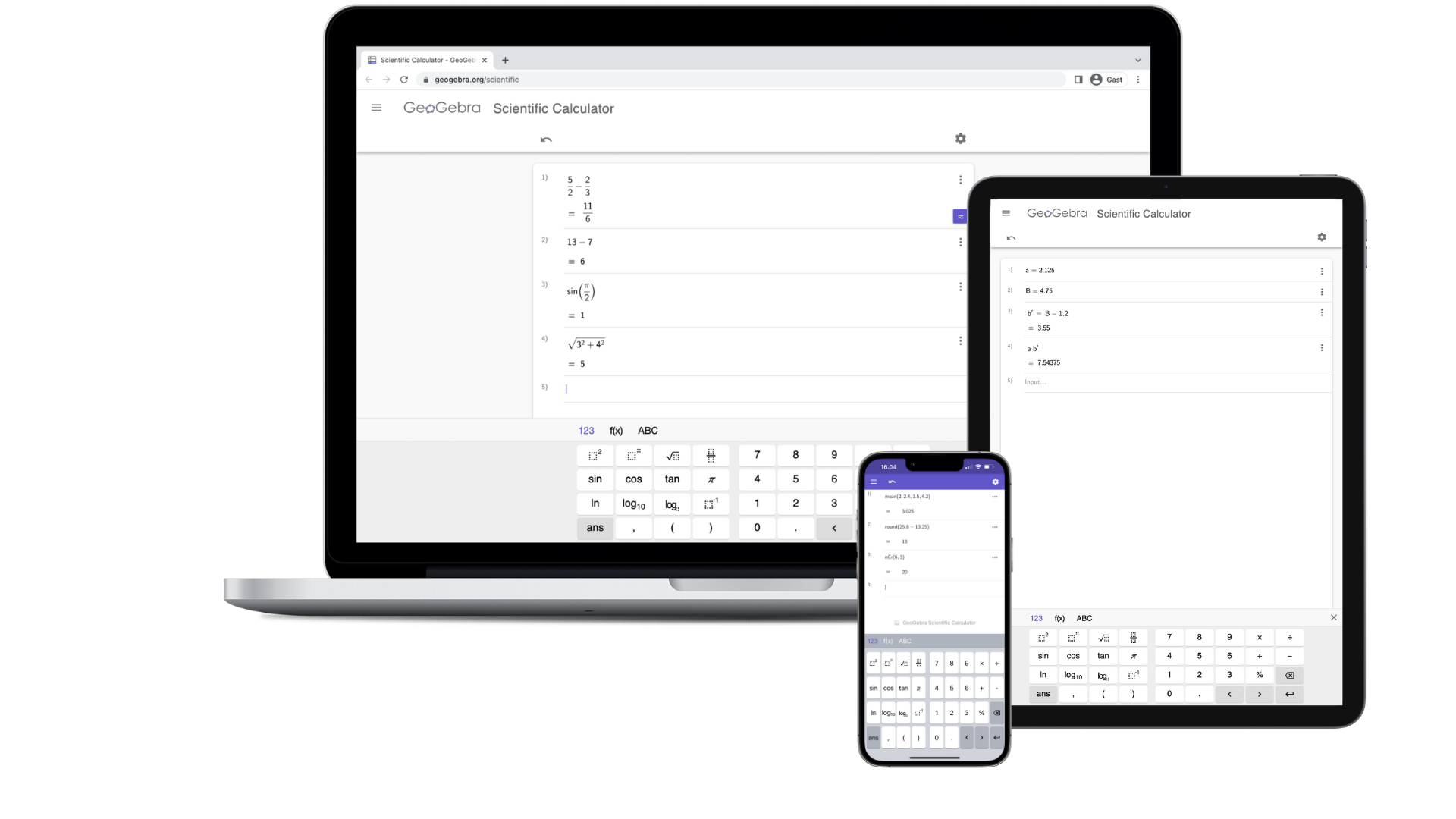# Scientific Calculator

## Introducing the Scientific Calculator

The GeoGebra Scientific Calculator is a powerful and user-friendly mathematics app. Say goodbye to your traditional calculator and embrace the simplicity and versatility of the GeoGebra Scientific Calculator. Designed to be accessible for users of all ages, even young students can effortlessly explore the exciting world of GeoGebra math apps.Here are the features of the GeoGebra Scientific Calculator:

• Computations with fractions
• Trigonometric functions: sin, cos, tan
• Statistical functions
• Exponential functions and logarithms

The Scientific Calculator offers these powerful features to enhance your mathematical calculations and explorations. Discover the full potential of the GeoGebra Scientific Calculator by exploring our official tutorial, complete with step-by-step instructions to guide you through its features and functionalities.